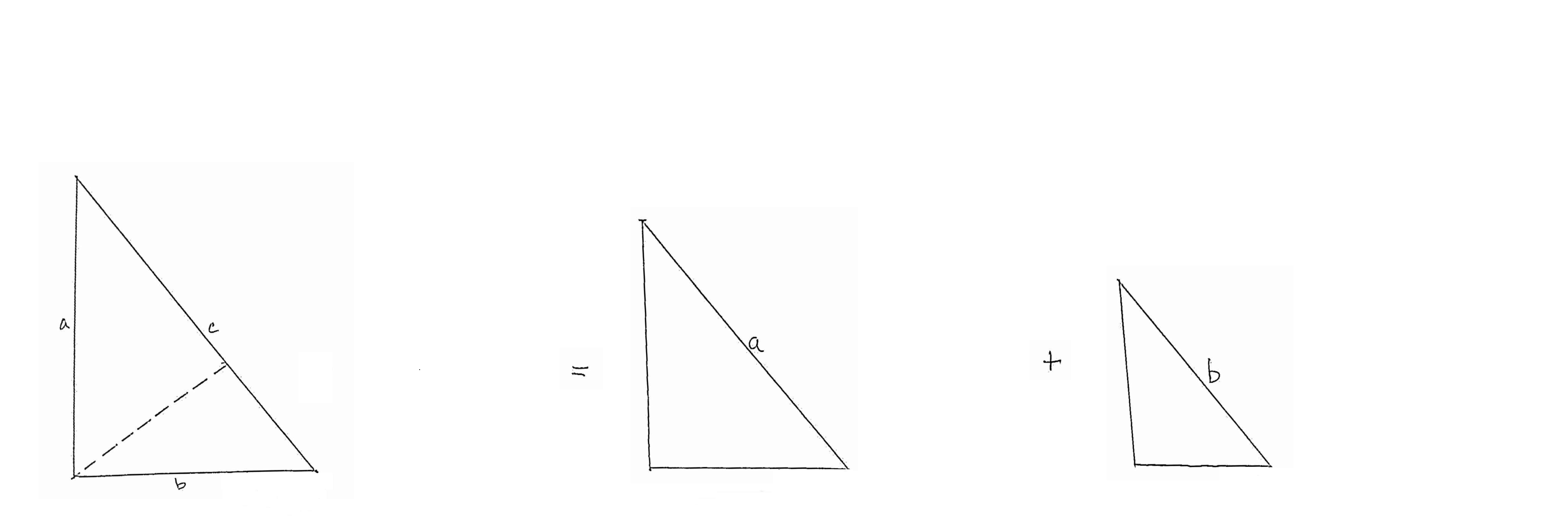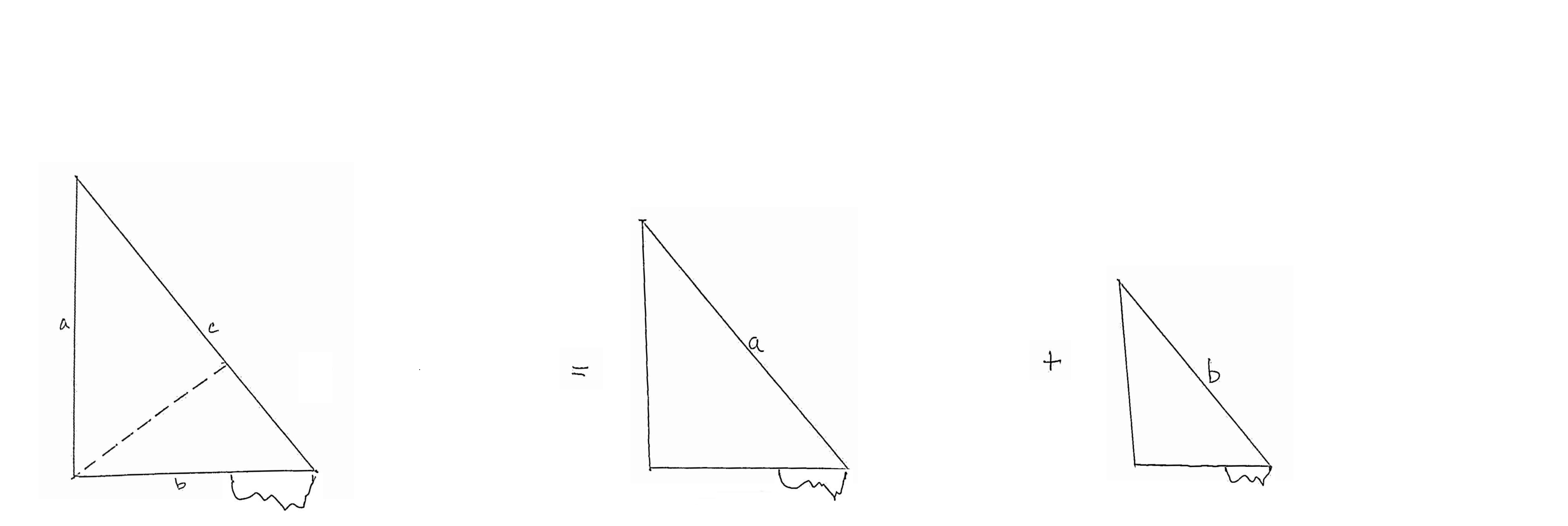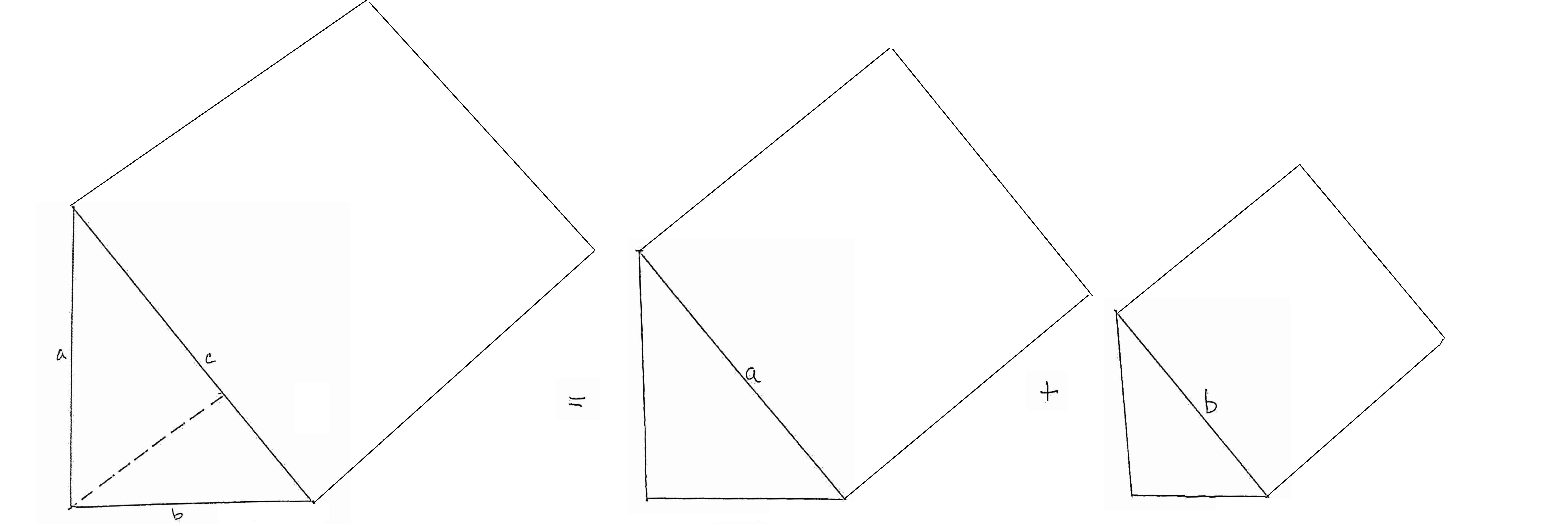Steve's new proof of the Pythagorean Theorem! Feb 3rd, 2014.  My homepage!

Feb 21: Oh no! I just discovered that Albert Einstein figured out this exact proof when he was 12. Since I am 5 times that age, my IQ must be 1/5th his.

Given: a right triangle, sides of length a and b, hypotenuse length c. Prove: c2 = a2 + b2.

Draw a line perpendicular to the hypotenuse and thru the opposite corner point. This line divides the triangle into two smaller triangles. To wit:Note that the two smaller trianges are both similar to each other and also similar to the original triangle. (Each shares a common angle and a right angle).

Note that the area of the original triangle equals the sum of the areas of the two new triangles (obviously, by construction).Now, let's draw some kind of a "bump" anywhere on the original triangle, of any arbitrary shape, and then draw a similar bump on both of the other triangles ("similar" in the rigorous sense, identical shape, proportionally sized at the same proportion as the triangle).

Claim: The area of any bump on the original triangle equals the sum of the areas of the similar bumps on the other two! That is:(This should also be "obvious": The sum of the areas of the two triangles equals the area of the larger one, by construction. And since they are all scaled by the same ratios, if it holds true for the triangles, it must also hold true for the bumps!)

Now, if you believe that simple truth, then the final step is quite trivial, and you have what I think is the world's most trivial, simple, and easy to understand proof ever of the Pythagorean Theorem.

Drum roll please.... I'm now gonna draw a very specific "bump" on all three triangles. Namely, a square on their hypotenuses:Do you get it already? QED!!

These squares are just big "bumps", no?

And we just said:
"The area of any bump on the original triangle equals the sum of the areas of the similar bumps on the other two!".
Thus, since a "square" is a "bump", and the above statement holds for any bump, then:
The area of the square on the hypotenuse of the original triangle equals the sum of the areas of the (similar) squares on the hypotenuses of the other two
QEF'nD: Quod erat f'n demonstrandum!

I'm gonna claim the above is the simplest proof of the Pythagorean Theorem ever devised! It is almost completely obvious by inspection. If you delete all text on this page and leave just the drawings, the sequence of figures almost proves itself! No algebra. No determination of the length of the perpendicular, or the relative lengths of the two pieces c is broken into. No nothin'!

In thinking more on this, I have come to this new idea, which I have not seen anywhere else. And it is this: is there any other 2D shape which can be cut with a single cut and produce two pieces which are similar to each other AND ALSO similar to the original? For example, cutting a rectangle in half produces two similar halves, but they are not similar to the original rectangle. Or cutting a square off of a golden rectangle produces one piece similar to the original but not to the other piece.

Thus, Swift's Right Triangle Hypothesis:
A right triangle is the ONLY 2D shape which can be cut with a single straight-line cut such that the two new pieces are both similar to each other and also similar to the original shape.
And THIS is what is unique about right triangles. This is the more fundamental truth from which the Pythagorean Theorem is merely a trivial corollary!